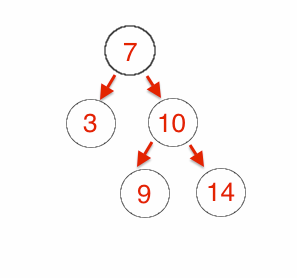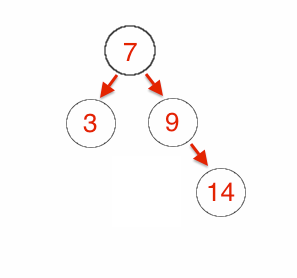## Monday, June 2, 2014

### Back To The Basics: The Binary Tree Deletion

In my previous post Back To The Basics: The Binary Tree Traversing I discussed several common algorithms for traversing a Binary Search Tree. In this post I’d like to turn our attention to one last common algorithm, delete.

The delete algorithm consists of three main scenarios. First, deleting a leaf node. Second deleting a node with only one child. Last, deleting a node with two children.

The first scenario is the easiest scenario to account for. Remember that a leaf node is a node which has no child nodes. So to delete a leaf node requires us simply to null out the value of that node in it's parent.The second scenario of deleting a node with only one child is also pretty straight forward. You can simply change the pointer that points to the node being deleted to point to the deleted node's child.

The third scenario is the more complicated one. When deleting a node with two children you replace the pointer from the node being deleted to either the minimum value node from the right tree or maximum value node from the left tree and then delete that node from that tree.Here is what that algorithm looks like.

public Tree<T> delete(T data) {
return this.delete(this, data);
}

private Tree<T> delete(Tree<T> parent, T data) {
if(parent == null)
return parent;

int comparison = data.compareTo(parent.data);
if(comparison < 0)
parent.left = this.delete(parent.left, data);
else if(comparison > 0)
parent.right = this.delete(parent.right, data);
else {
if(parent.right == null)
return parent.left;
if(parent.left == null)
return parent.right;

Tree<T> temp = parent;
parent = temp.right.minTree();
parent.right = this.deleteMin(temp.right);
parent.left = temp.left;
}

return parent;
}

private Tree<T> deleteMin(Tree<T> parent) {
if(parent.left == null)
return parent.right;
return this.deleteMin(parent.left);
}